# Betty's Balloons

Betty blows up 14 balloons. Betty gives 7 balloons to Ben. How many balloons does Betty still have? Show all your mathematical thinking.

Assessment

## Plan

#### Formal Mathematical Language and Symbolic Notation

A student may independently select a printed number line, number chart, ten frames, graph paper, etc. as they work on a task.

### Suggested materials

Engagement Image:

Teachers may project the image below to launch this task for their students, define nouns, promote discussion, access prior knowledge, and inspire engagement and problem solving.

## Solutions tabs

### Expert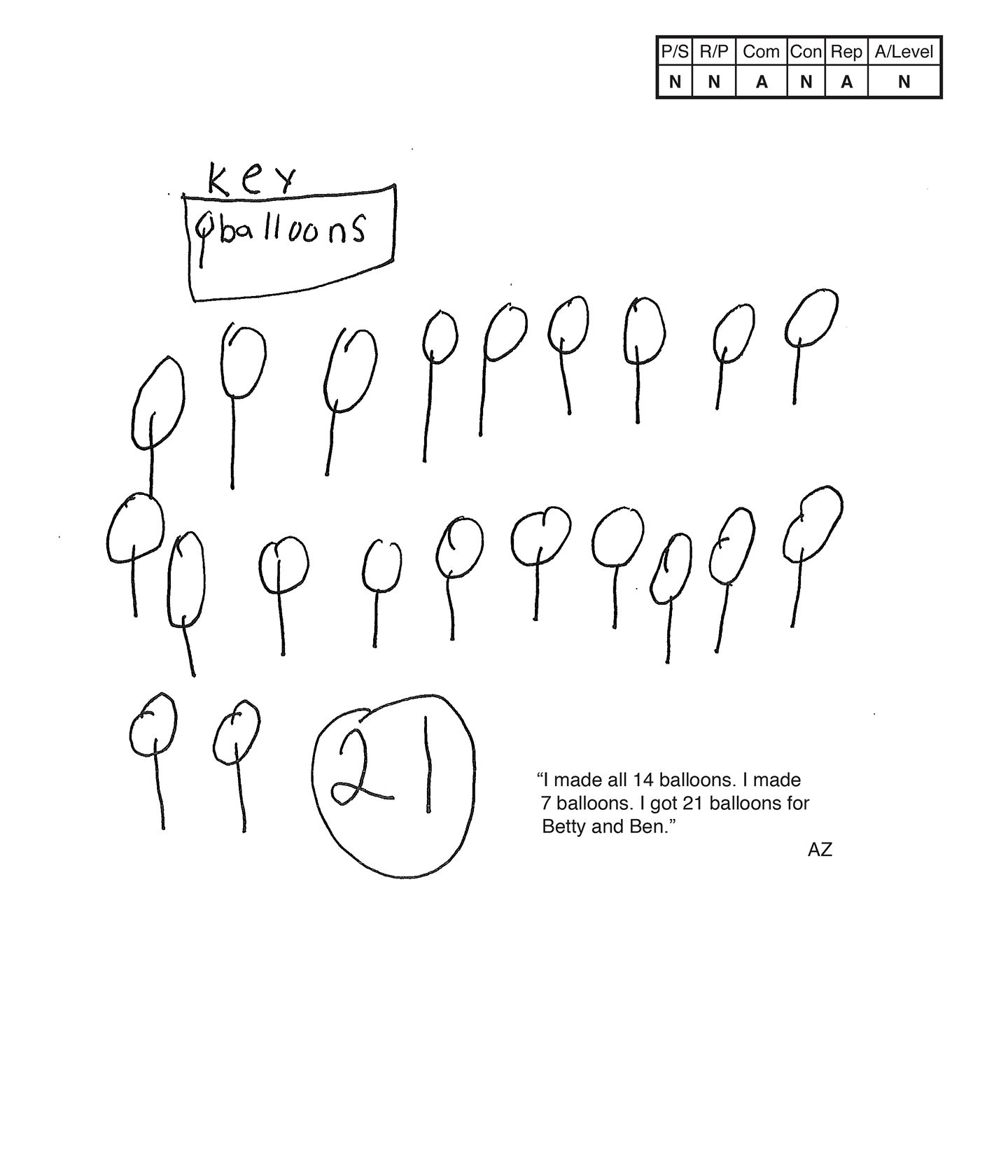This student does not meet the standard.

### Scoring Rationale

#### Novice

The student's strategy of making a diagram of fourteen balloons and seven balloons and totaling them for a sum of twenty-one does not work to solve the task. The student's answer, "21," and, "I got 21 balloons for Betty and Ben," is not correct.

#### Novice

The student's solution does not demonstrate understanding of the underlying mathematics of the task. The student does not use the concept of subtraction to determine that Betty would have seven balloons remaining after giving seven balloons to Ben. The teachers scribes, "I made all 14 balloons. I made 7 balloons, I got 21 balloons for Betty and Ben." This does not support correct reasoning.

#### Apprentice

The student correctly uses the mathematical term key.

#### Novice

The student does not include a connection in their solution.

#### Apprentice

The student attempts a diagram but it is not accurate. The student provides a key for balloons. It appears that the student diagrams fourteen balloons to match the number in the first sentence and then seven balloons to match the number in the second sentence and totals them to find twenty-one balloons.

#### Novice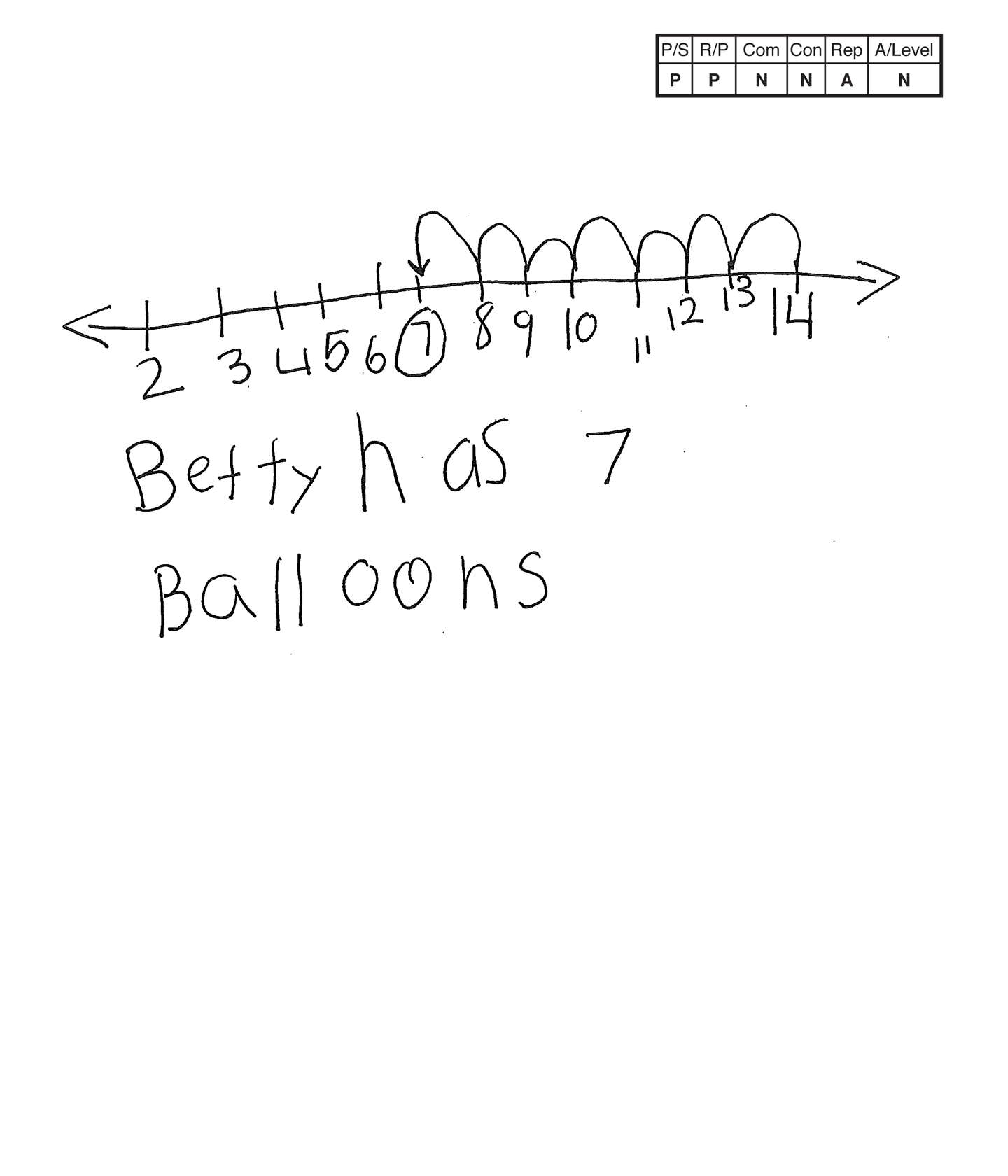This student does not meet the standard.

### Scoring Rationale

#### Practitioner

The student's strategy of making a number line to show fourteen balloons and counting back to seven balloons would work to solve this task. The student's answer, "Betty has 7 Balloons," is correct.

#### Practitioner

Arguments are constructed with adequate mathematical basis. The student's text supports the number line.

#### Novice

The student does not use any mathematical language. Scribing for the student could have provided mathematical language.

#### Novice

The student does not include a connection in their solution.

#### Apprentice

The student's number line is appropriate but not accurate. The student omits the number one and does not provide a label for balloons.

#### Novice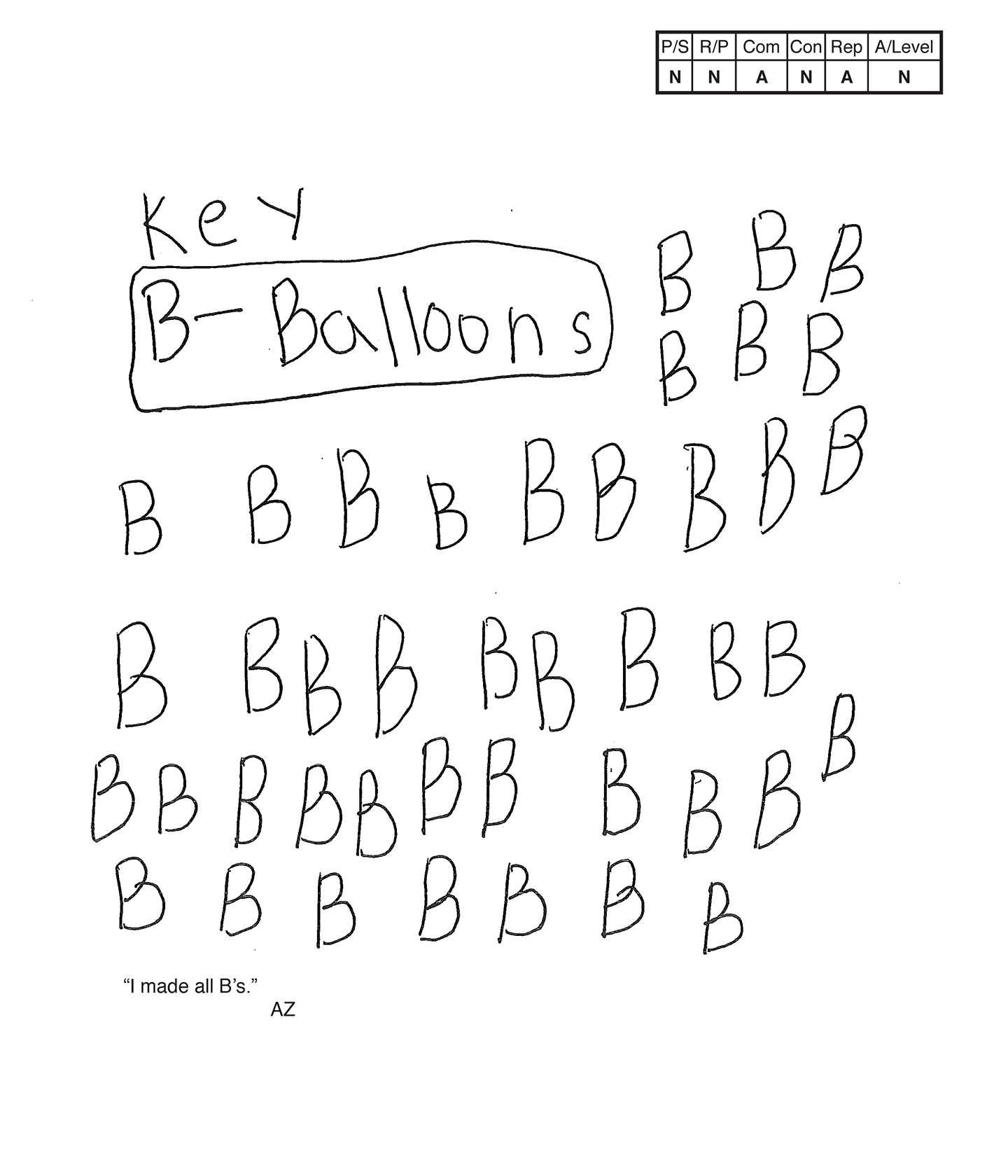This student does not meet the standard.

### Scoring Rationale

#### Novice

The student's strategy of filling their paper with the letter B does not work to solve the task. The student does not state an answer.

#### Novice

The student's solution does not demonstrate understanding of the underlying mathematics of the task. The student does not use the concept of subtraction to determine that Betty would have seven balloons remaining after giving seven balloons to Ben. The student's statement, "I made all B's," does not support correct thinking.

#### Apprentice

The student correctly uses the mathematical term key. The student is given credit for the term because they do use the "B" in their solution to represent balloons.

#### Novice

The student does not include a connection in their solution.

#### Apprentice

The student attempts a diagram by making the letter B for balloons but seems to simply fill their paper with the letter and stating, "I made all B's."

#### Novice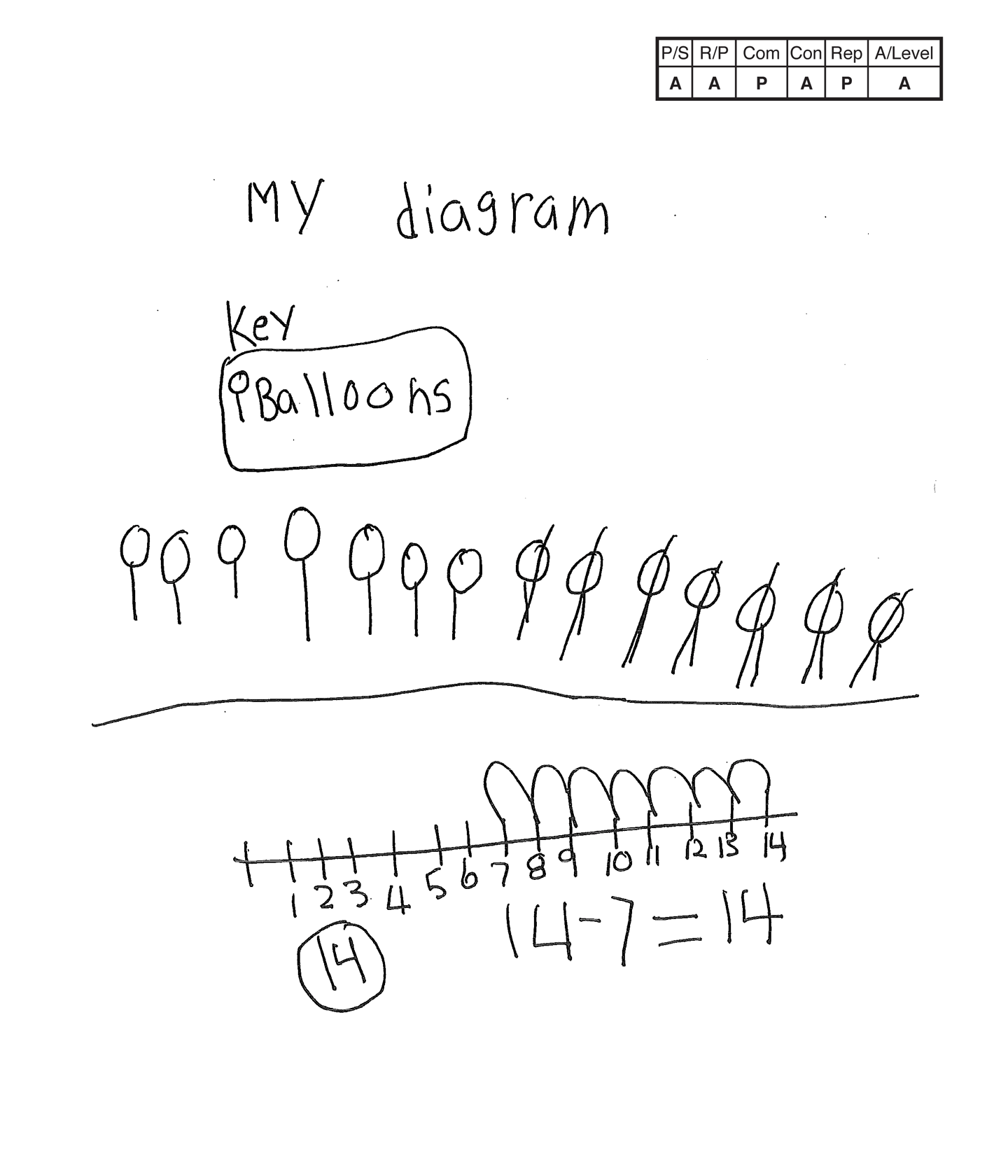This student does not meet the standard.

### Scoring Rationale

#### Apprentice

The student's strategy of making a diagram to show fourteen balloons and counting back by crossing out seven balloons would work to solve this task. However, the student does not state an answer.

#### Apprentice

The student's diagram and number line show correct reasoning. The student is able to represent fourteen balloons and count back seven balloons. The diagram and the number line indicate an answer of seven balloons, but the student does not state an answer. The student sets up an appropriate equation, but the subtraction is incorrect.

#### Practitioner

The student correctly uses the mathematical terms diagram, key.

#### Apprentice

The student attempts a new strategy, but their answer, "14," does not support the number line.

#### Practitioner

The student's diagram is appropriate and accurate with a key to define the balloons. The student's number line lacks a label to identify the numbers as representing balloons. Only one representation needs to be appropriate and accurate to earn a Practitioner score.

#### Apprentice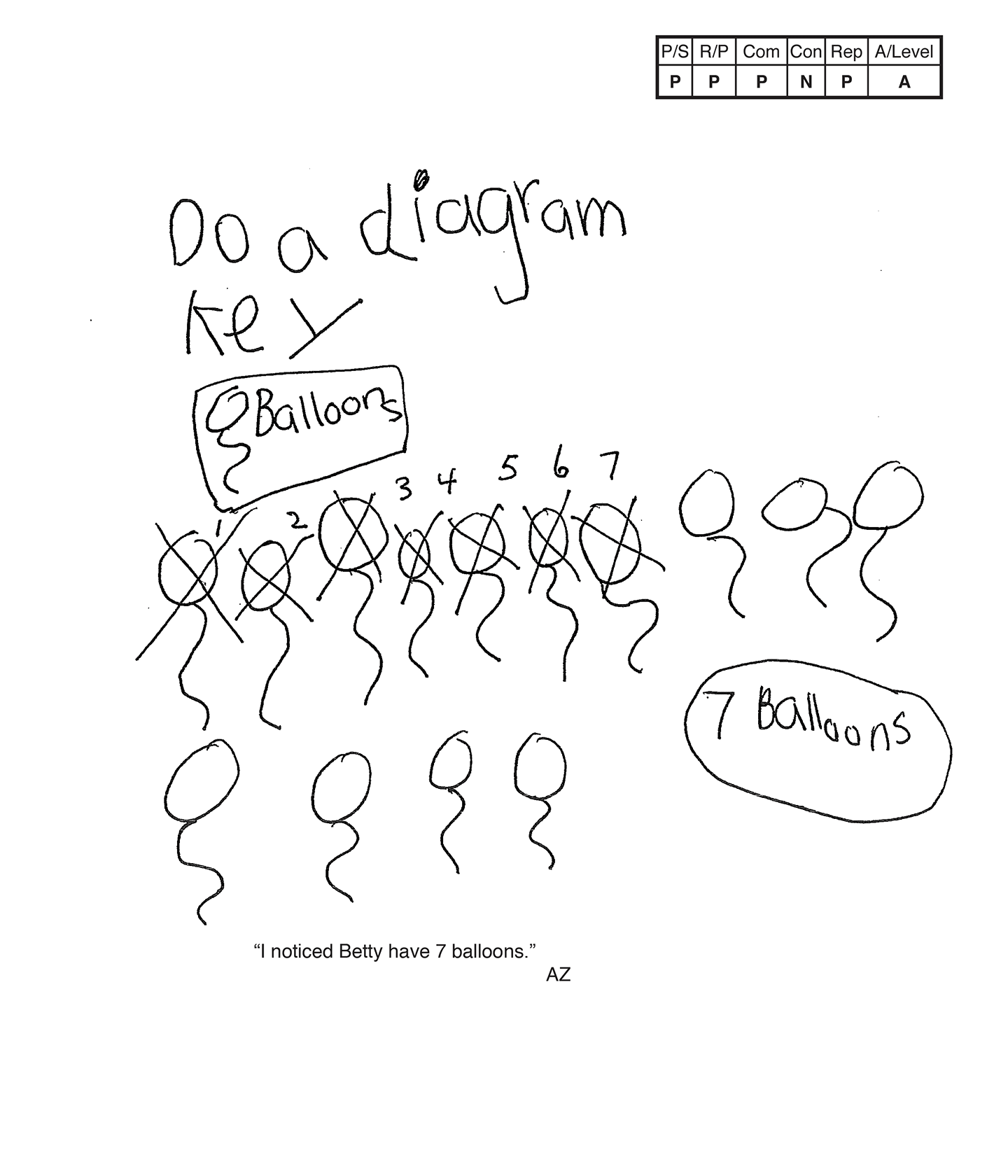This student does not meet the standard.

### Scoring Rationale

#### Practitioner

The student's strategy of making a diagram to show fourteen balloons and counting forward while crossing out seven balloons works to solve this task. The student's answer, "7 Balloons," is correct.

#### Practitioner

The student's argument is constructed with adequate mathematical basis. The diagram supports the student's correct reasoning.

#### Practitioner

The student correctly uses the mathematical terms diagram, key.

#### Novice

The student does not attempt a mathematical connection. "I noticed Betty have 7 balloons," is a restating of the answer.

#### Practitioner

The student's diagram is appropriate and accurate with a key to define the balloons.

#### Apprentice

Note:

The overall achievement level for this piece of student work falls under Exemplars exception to the rule category. If a student has all Apprentice scores or above, but a Novice in "Connections," the student may still receive an achievement level score of Apprentice. To learn more about Exemplars scoring, please refer to the section of your dashboard called "Tools for Success" and click on the link for “Using the Assessment Rubric.”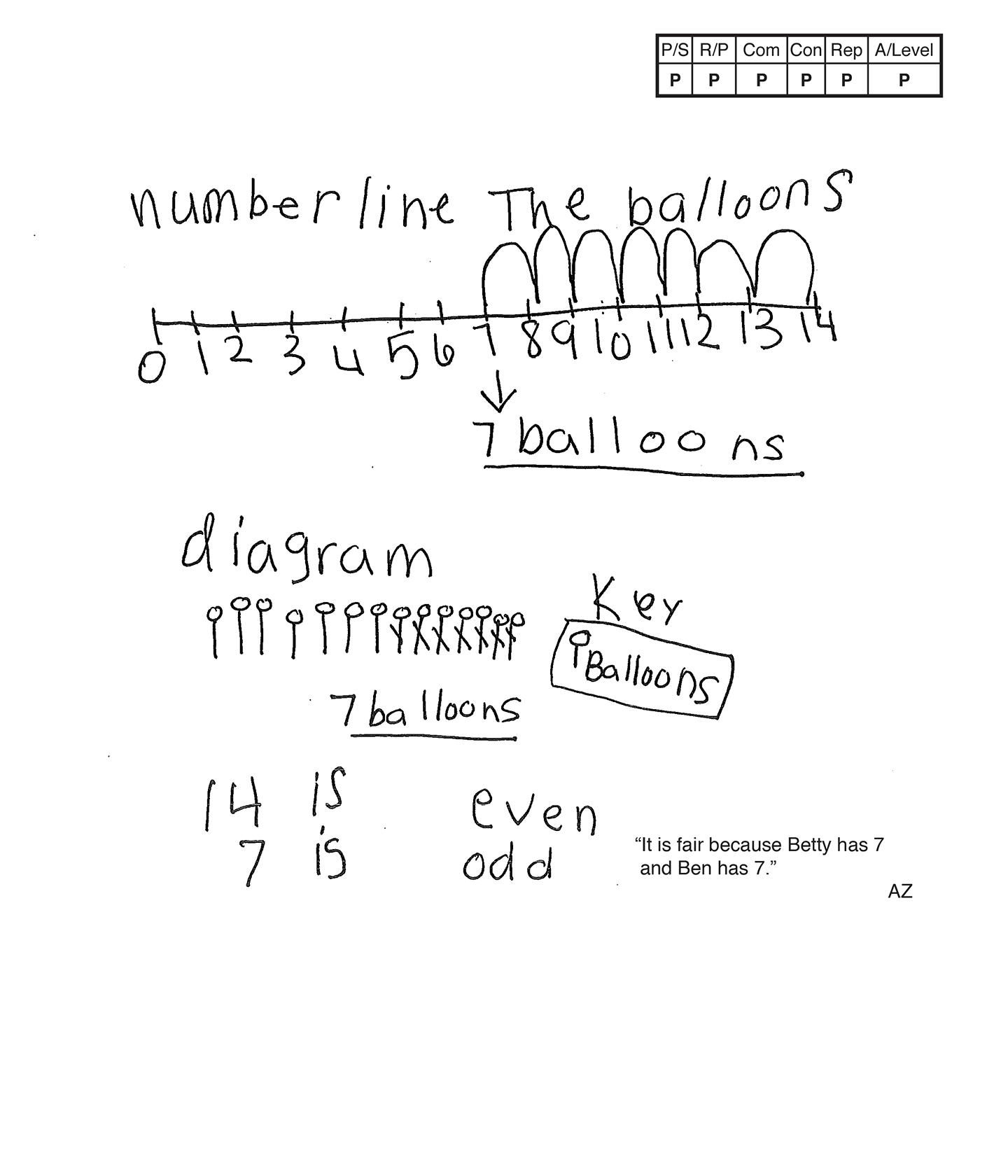This student meets the standard.

### Scoring Rationale

#### Practitioner

The student's strategy of making a number line to show fourteen balloons and counting back seven balloons works to solve this task. The student's answer, "7 balloons," is correct.

#### Practitioner

The student's argument is constructed with adequate mathematical basis. The number line, diagram and text support the student's correct reasoning.

#### Practitioner

The student correctly uses the mathematical terms number line, diagram, key, even, odd.

#### Practitioner

The student uses an alternative strategy of a diagram to show 7 balloons for Betty. The student does not use the diagram to verify their number line for an Expert connection. The student makes the mathematically relevant observations, "14 is even, 7 is odd," and, "It is fair because Betty has 7 and Ben has 7."

#### Practitioner

The student's number line is appropriate and accurate. The title defines the numbers as representing balloons. The student's diagram is appropriate and accurate with a key to define the balloons.

#### Practitioner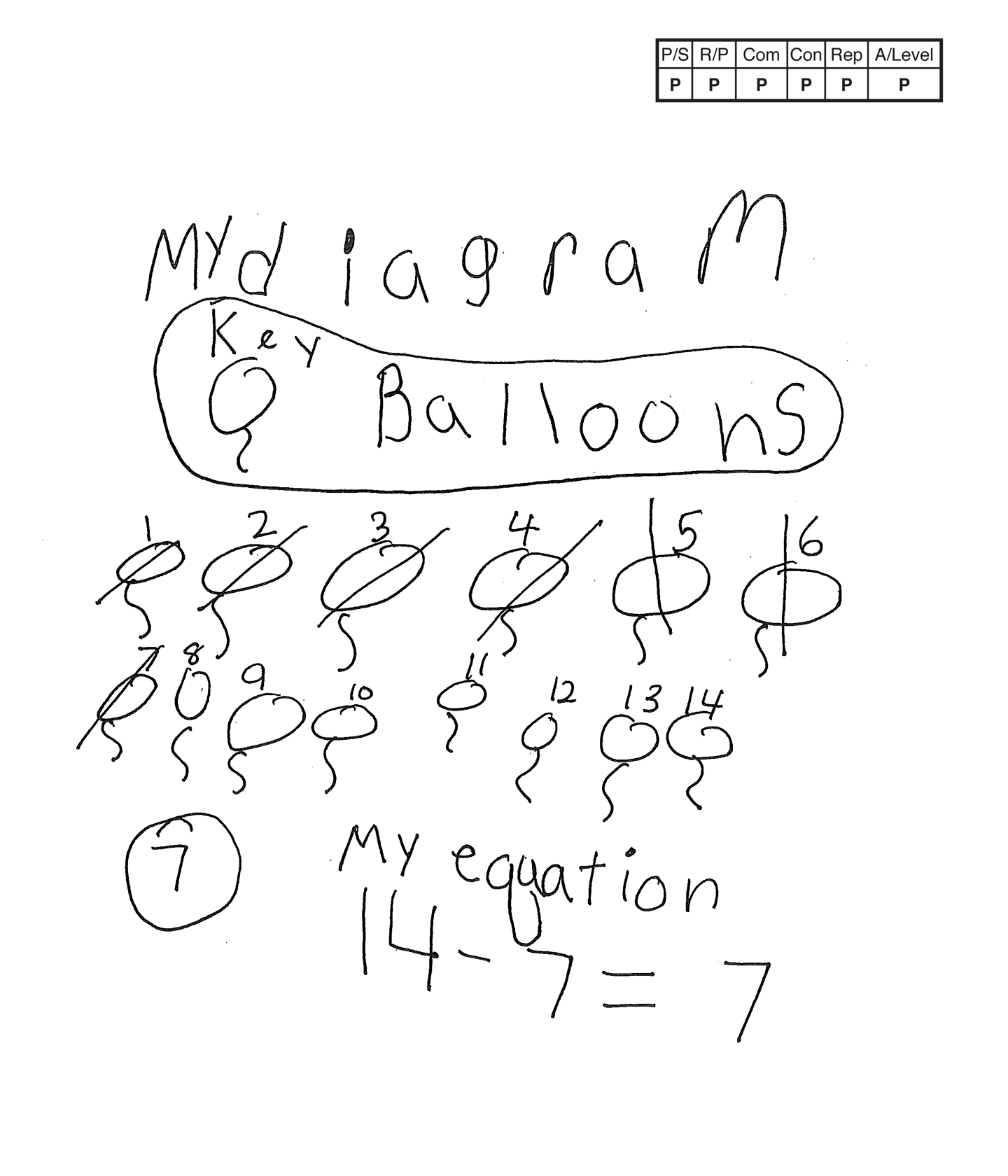,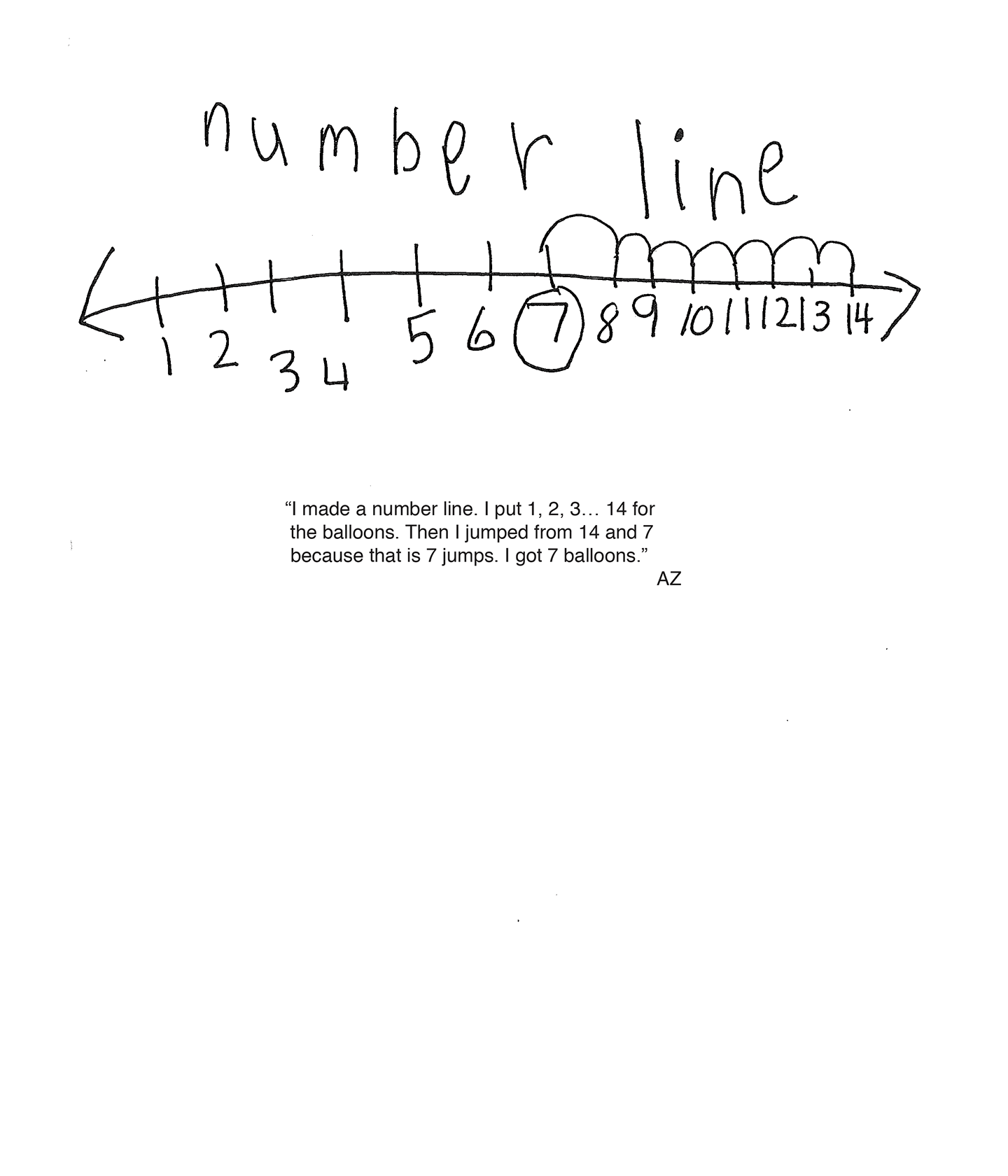This student meets the standard.

### Scoring Rationale

#### Practitioner

The student's strategy of making a diagram to show fourteen balloons and counting forward crossing out seven balloons works to solve this task. The student's answer, "7," is correct.

#### Practitioner

The student's argument is constructed with adequate mathematical basis. The diagram, equation, number line and scribing support the student's correct reasoning.

#### Practitioner

The student correctly uses the mathematical terms diagram, key, equation, number line.

#### Practitioner

The student uses an equation and a number line to show Betty having seven balloons remaining after giving seven balloons to Ben. The student could have reached an Expert level if they had linked the three strategies together and stated that the answer was always seven balloons.

#### Practitioner

The student's diagram is appropriate and accurate with a key to define the balloons for Betty and Ben. The student's number line is appropriate and accurate. The scribing provides a label to define the "balloon numbers."

#### Practitioner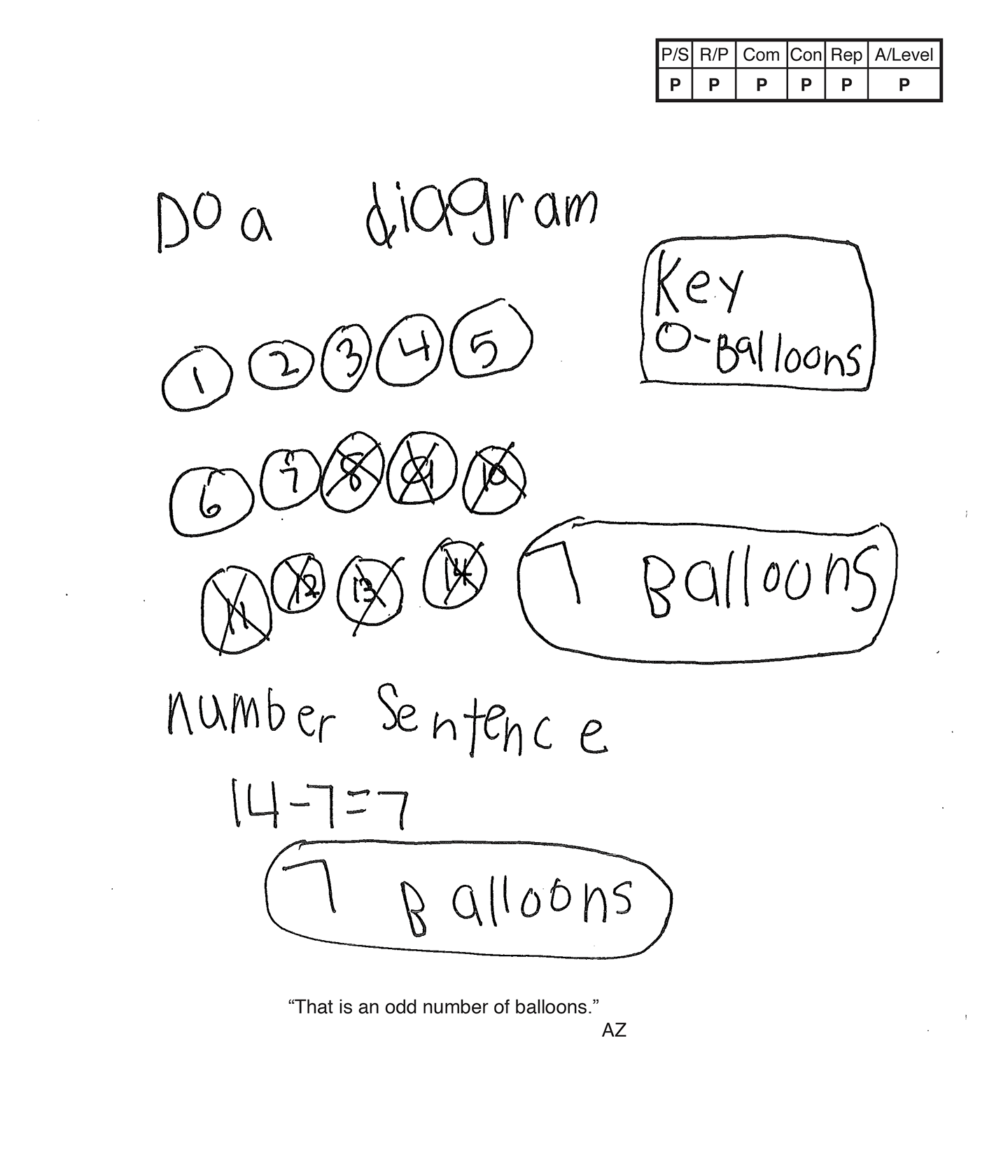This student meets the standard.

### Scoring Rationale

#### Practitioner

The student's strategy of making a diagram to show fourteen balloons, crossing out seven balloons, works to solve this task. The student's answer, "7 balloons," is correct.

#### Practitioner

The student's argument is constructed with adequate mathematical basis. The diagram, equation and scribing support the student's correct reasoning.

#### Practitioner

The student correctly uses the mathematical terms key, diagram, odd.

#### Practitioner

The student uses a "number sentence," "14 – 7 = 7," to show how many balloons Betty has and states, "7 Balloons." The student does not earn an Expert connection for verification because they do not connect the equation to the diagram. The student makes the relevant observation, "That is an odd number of balloons."

#### Practitioner

The student's diagram is appropriate and accurate with a key to define the balloons.

#### Practitioner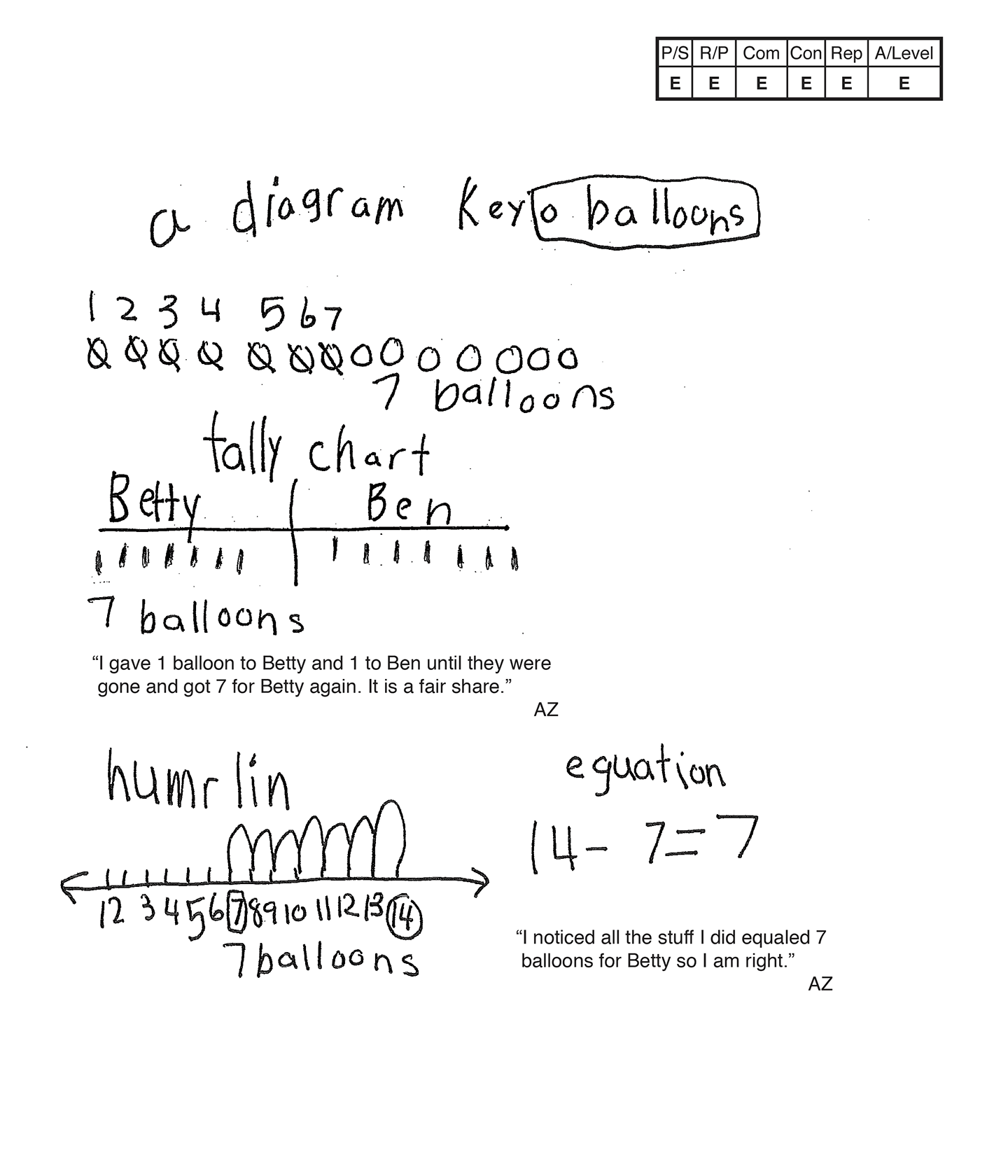This student exceeds the standard.

### Scoring Rationale

#### Expert

The student's strategy of making a diagram to show fourteen balloons and counting forward crossing out seven balloons works to solve this task. The student's answer, "7 balloons," is correct. The student verifies their answer by using alternative strategies.

#### Expert

The student's argument is constructed with adequate mathematical basis. The diagram, tally chart, number line, equation, and scribing justify and support the student's correct reasoning.

#### Expert

The student correctly uses the mathematical terms diagram, key, tally chart, "numrlin," "eguation," odd, even, equaled.

#### Expert

The student makes the Practitioner observations, "I noticed 7 is odd and 14 is even," and, "I gave 1 balloon to Betty and 1 to Ben until they were gone and got 7 for Betty again. It is a fair share." The student makes an Expert connection. The student uses a tally chart, number line and an equation to verify that seven balloons is a correct answer and states, "I noticed all the stuff I did equaled 7 balloons for Betty so I am right."

#### Expert

The student's diagram is appropriate and accurate with a key to define the balloons. The student's tally chart is accurate with all necessary labels provided. The student's number line is appropriate and accurate with the balloon numbers labeled.